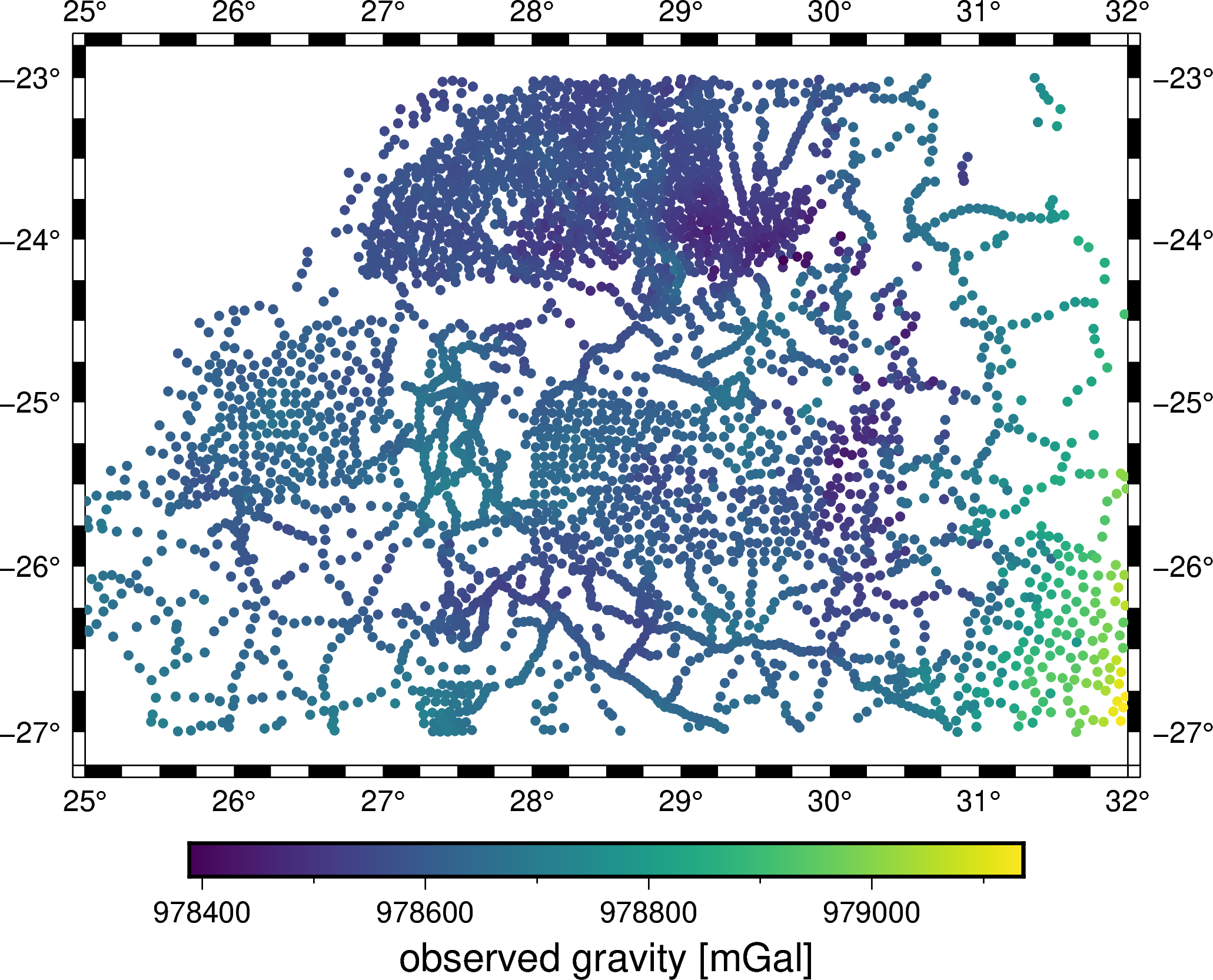# Live demo: Processing gravity data with Fatiando a Terra¶

This notebook is based on the Harmonica tutorial at Transform 2021.

## Import packages¶

In :
# The standard Python science stack
import numpy as np
import pandas as pd
import xarray as xr

# For projections (wrapped for Proj)
import pyproj

# Plotting maps using GMT
import pygmt

# The Fatiando stack
import pooch
import verde as vd
import boule as bl
import harmonica as hm


## Data from the Bushveld Igneous Complex (South Africa)¶

We can use Pooch to download data files from anywhere on the web. Let's download some public domain gravity data from the Bushveld Igneous Complex that we have on some GitHub repositories.

In :
url_grav = "https://github.com/fatiando/2021-gsh/raw/main/data/bushveld_gravity.csv"
md5_grav = "md5:45539f7945794911c6b5a2eb43391051"

url_topo = "https://github.com/fatiando/transform21/raw/main/data/bushveld_topography.nc"
md5_topo = "md5:62daf6a114dda89530e88942aa3b8c41"

In :
path_grav = pooch.retrieve(url_grav, known_hash=md5_grav)
path_topo = pooch.retrieve(url_topo, known_hash=md5_topo)

print(path_grav)
print(path_topo)

/home/leo/.cache/pooch/f9087e49a3b8e83d4e2affba9882d9ad-bushveld_gravity.csv
/home/leo/.cache/pooch/da3d295d727d76ff80f092a51df3d2fa-bushveld_topography.nc


Use Pandas to read the gravity data from the CSV file.

In :
data = pd.read_csv(path_grav)
data

Out:
latitude longitude elevation gravity
0 -26.26334 25.01500 1230.16 978681.38
1 -26.38713 25.01932 1297.00 978669.02
2 -26.39667 25.02499 1304.84 978669.28
3 -26.07668 25.04500 1165.24 978681.08
4 -26.35001 25.07668 1262.47 978665.19
... ... ... ... ...
3872 -23.86333 31.51500 300.53 978776.85
3873 -23.30000 31.52499 280.72 978798.55
3874 -23.19333 31.54832 245.67 978803.55
3875 -23.84833 31.57333 226.77 978808.44
3876 -23.00000 31.37500 285.59 978734.77

3877 rows × 4 columns

Use xarray to read the topography data from the netCDF file.

In :
topography = xr.load_dataarray(path_topo)
topography

Out:
<xarray.DataArray 'bedrock' (latitude: 240, longitude: 419)>
array([[1257., 1260., 1266., ...,  195.,  201.,  425.],
[1245., 1254., 1261., ...,  206.,  215.,  375.],
[1256., 1258., 1268., ...,  200.,  232.,  300.],
...,
[1029., 1031., 1033., ...,  248.,  242.,  238.],
[1029., 1031., 1033., ...,  247.,  242.,  237.],
[1028., 1030., 1032., ...,  247.,  241.,  238.]])
Coordinates:
* longitude  (longitude) float64 25.02 25.03 25.05 25.07 ... 31.95 31.97 31.98
* latitude   (latitude) float64 -27.0 -26.98 -26.97 ... -23.05 -23.03 -23.02
Attributes:
long_name:       Bedrock relief
actual_range:    [-10898.   8271.]
units:           meters
vertical_datum:  sea level
datum:           WGS84

Use pygmt to plot the data.

In :
fig = pygmt.Figure()
pygmt.makecpt(cmap="viridis", series=[data.gravity.min(), data.gravity.max()])
fig.plot(
x=data.longitude,
y=data.latitude,
color=data.gravity,
cmap=True,
style="c4p",
projection="M15c",
frame=True,
)
fig.colorbar(frame='af+l"observed gravity [mGal]"')
fig.show()

Out:In :
fig = pygmt.Figure()
pygmt.makecpt(cmap="earth", series=[topography.values.min(), topography.values.max()])

grdinfo [WARNING]: The x-coordinates and range attribute are in conflict but range is exactly 360; we rely on this range